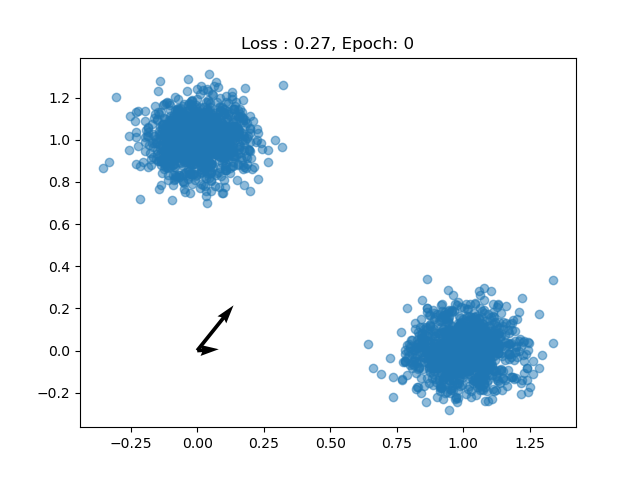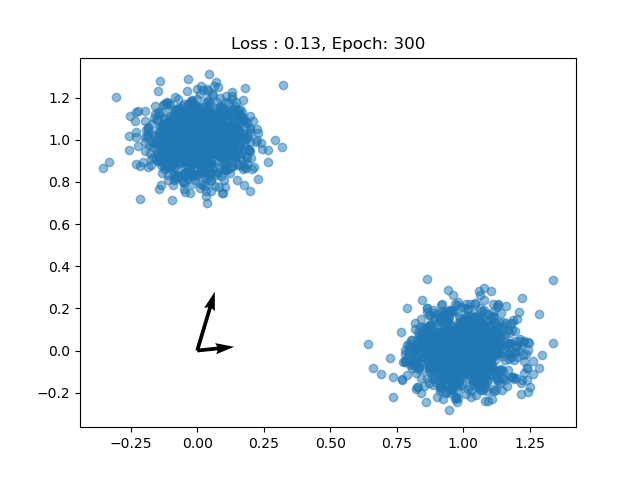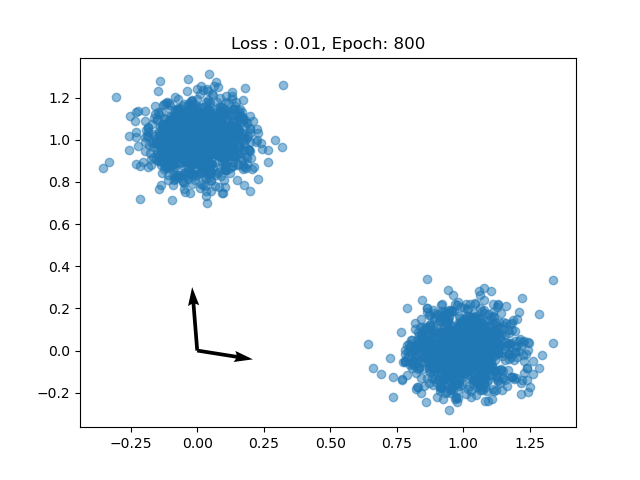Dictionary learning is a popular method for building sparse representations of a dataset. Given a dataset $X = [x_1, x_2, .., x_N]$ where $x_i \in \mathbb{R}^d$, we say that a dictionary is a set of vectors $D = [d_1, d_2,.., d_M]$ where $d_i \in \mathbb{R}^{d}$ so that it lies in the same space as the original data. There are various choices of dictionary, but in general we want one that encodes the original data with the smallest amount of lost information. For that purpose, we define the following criterion given a dictionary and a dataset:

$|| X - DR ||_2^2$

where R can be thought of soft assignment of each point each dictionary term. Thus, $R \in \mathbb{R}^{M}$ and tells us to which degree each data point belongs to each term. With this, we have a representation for each data point. To ensure that the representation will be sparse, we typically add a term to the loss which penalizes the norm of the representation vectors. One can also add a term that pushes dictionary terms to be orthogonal. In our case, the model that outputs representations will have a Softmax layer so we will guarantee sparsity by construction.

There are various methods for solving for $D$ and $R$ given the above criterion. Here, I will show a method done with gradient descent and implemented in PyTorch.

First let’s build a toy dataset, I’ll choose a 2 dimensional multivariate gaussian with two distinct components.

import matplotlib.pyplot as plt
from numpy.random import multivariate_normal as mn
import torch

data_1 = torch.tensor(mn([1, 0], [[.01, 0], [0, .01]], 1000), dtype=torch.float)
data_2 = torch.tensor(mn([0, 1], [[.01, 0], [0, .01]], 1000), dtype=torch.float)

data = torch.cat([data_1, data_2])

plt.plot(data[:,0], data[:,1])
plt.show()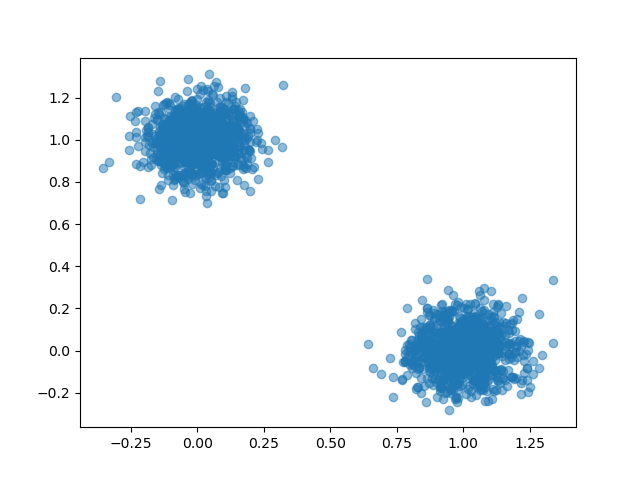Now we build a model that has two main components: a parameter matrix which holds our dictionray terms, and an attributor which in this case will be a simple linear model.

class DictionaryLearning(torch.nn.Module):

def __init__(self, dim, n_terms):
super(DictionaryLearning, self).__init__()
self.dim = dim
self.n_terms = n_terms
self.dictionary = torch.nn.Parameter(torch.rand((n_terms, dim),
dtype=torch.float,
))

self.attributor = self.build_attributor()

def build_attributor(self):
layers = torch.nn.ModuleList()
layers.append(torch.nn.Linear(self.dim, self.n_terms))
layers.append(torch.nn.Softmax())

def forward(self, x):
return self.attributor(x)

pass



Now we can train the model. We know that the data has two components so we’ll choose 2 dictionary terms. But different choices of $M$ can have different interpretations.

epochs = 10000
n_terms = 2
dim = 2

model = DictionaryLearning(dim, n_terms)

for e in range(epochs):

loss.backward()
optimizer.step()


We can plot the loss at every epoch to make sure the model is learning: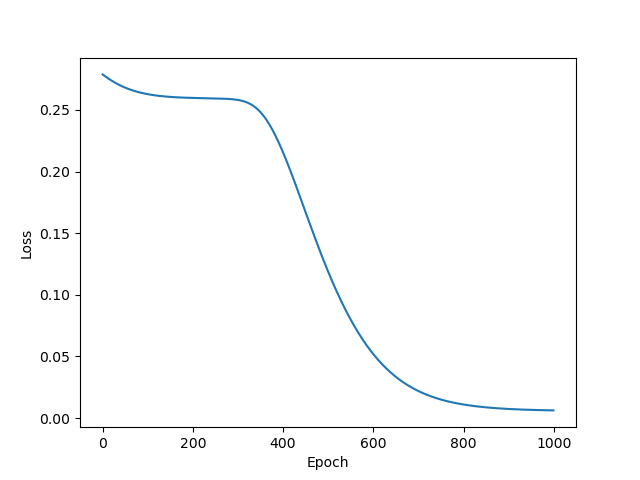And after every couple of epochs we can see the dictionary terms moving toward the data.